# Tensor basic definitionDRAFT NOTES

It's best to have prior knowledge about: matrix, matrix multiplication, vector matrix operations, representing transformations and such

# Easy but incomplete definition

## I - Tensor = multi-dimensional array of numbers

A tensor is often thought of as a generalized matrix. That is, it could be a 1-D matrix (i.e. a vector), a 3-D matrix (a cube of numbers), a 0-D matrix (a single number), or a higher dimensional structure that is harder to visualize.

The 'n' of n-D in this case is called the order, degree or rank of the tensor.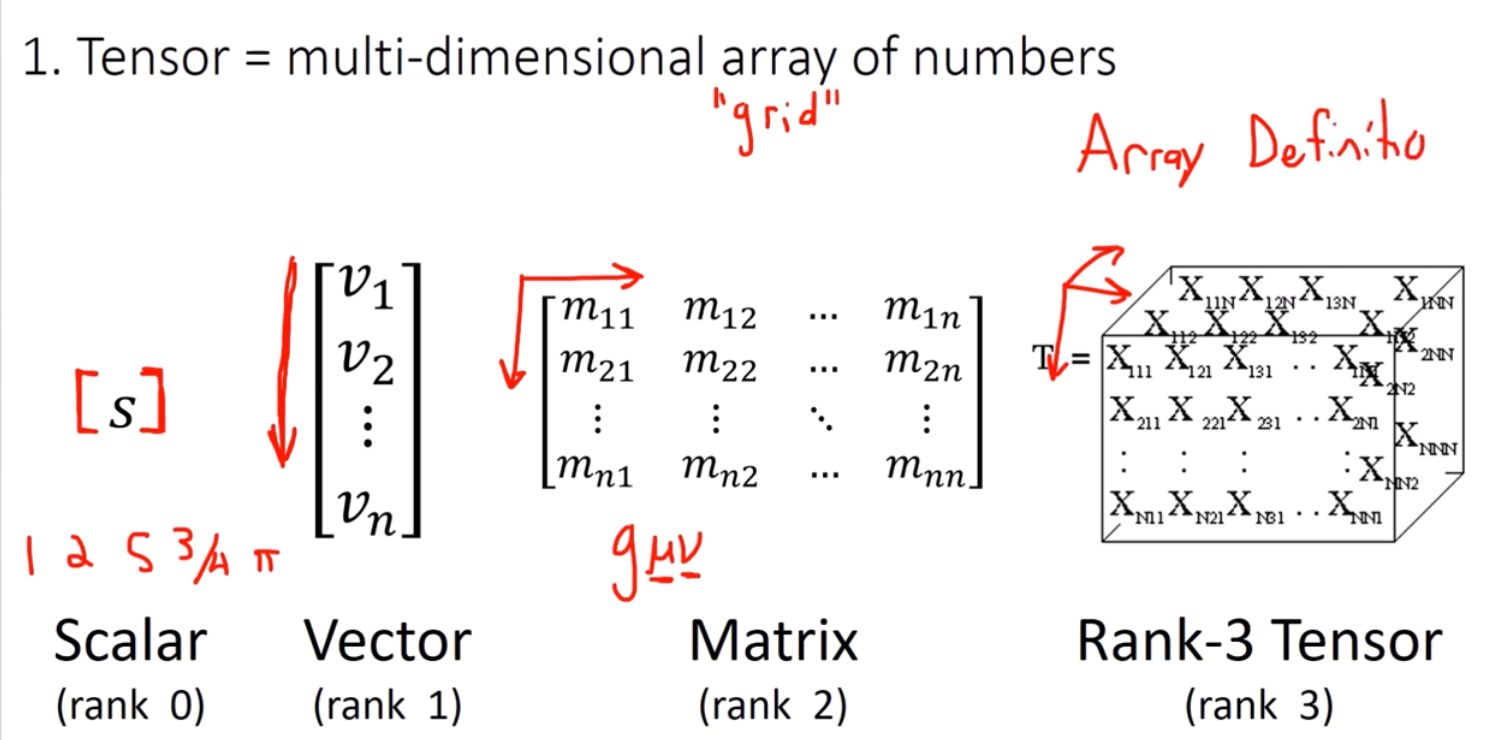## Examples of rank 2 tensors

Electromagnetic tensor: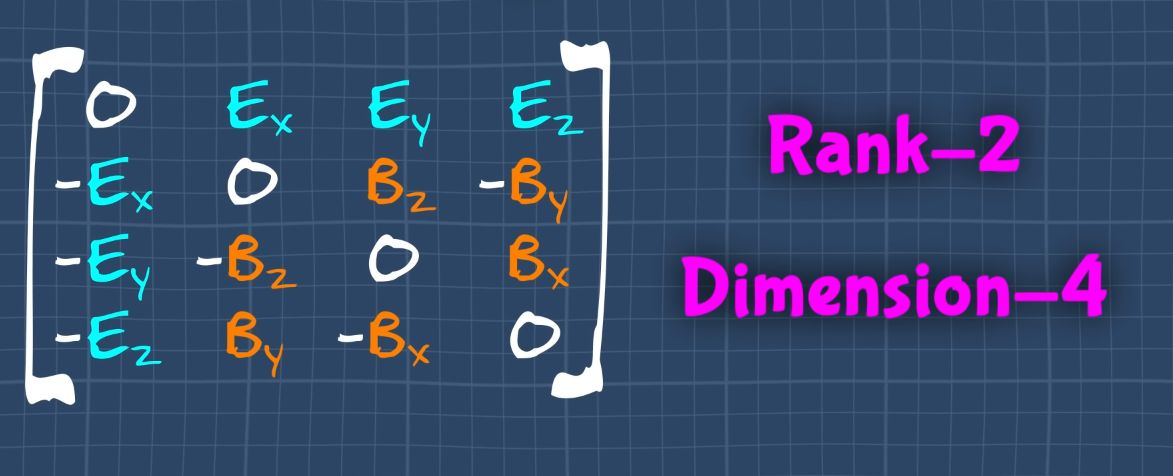Stress tensor:Rank 3, dimension - 3 (i.e 3 elements along the rows and columns)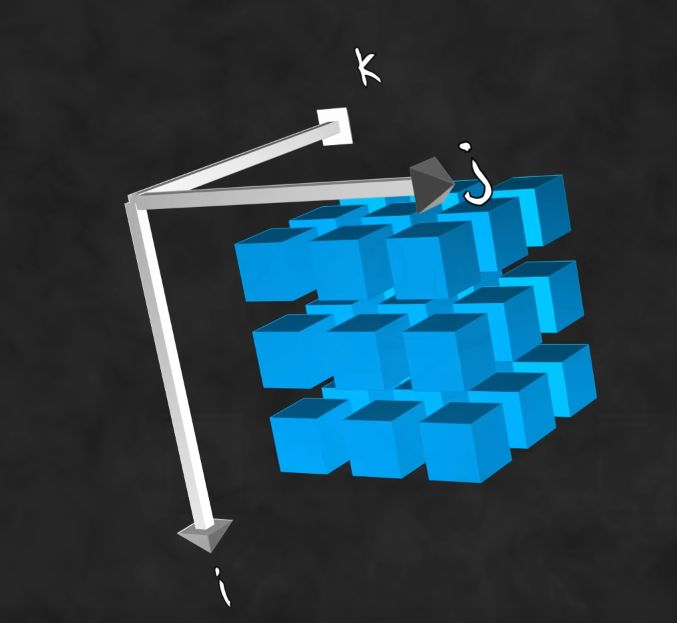Rank 4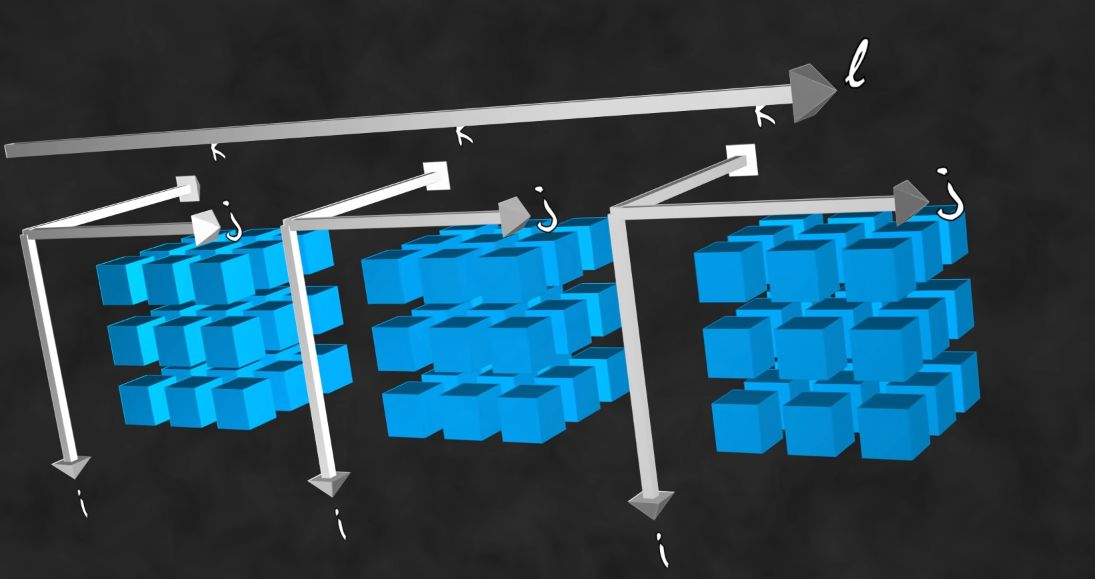### Index notation

Each element (number) position in the tensor can be described using indices:

• Rank-0: $$\tau$$, a scalar
• Rank-1: $$\tau_i$$, a vector
• Rank-2: $$\tau_{ij}$$, a matrix (Latin letters for Dimension-2/3)
$$\tau_{\alpha\beta}$$ (Greek letters for Dimension-4)
• Rank-3: $$\tau_{ijk}$$, a cube of matrices.
• Rank-4: $$\tau_{ijkl}$$, an array of cubes
• ...

# 3D Stress tensor

So far in our description it might be hard to see the need to call this a "tensor", why not just call it a matrix or multidimensional array of numbers? Well, when using the term "Tensor" the terminology indicates us how to interpret this object and what rules should apply (what operations are allowed). To be a bit more concrete: it's a difference somewhat similar to calling "3D vector" what we could simply describe as a "3x1 matrix". While a 3x1 matrix is just a generic array of numbers. It could represent temperatures in 3 different cities, the age of 3 persons for instance. When using the term "vector" we usually expect it to represent a direction in space. Likewise a "tensor" is a specific terminology used in the context of physics and continuum mechanics.

So let's see a very concrete example of a rank-2 tensor a "stress tensor". Somewhat similar to a transformation matrices, stress tensors will store a stress force at each row of the tensor (i.e. 3x3 matrix), We will name those stress forces $$\vec X$$, $$\vec Y$$ and $$\vec Z$$:

$T_{ij} = \begin{bmatrix} \vec X \\ \vec Y \\ \vec Z \\ \end{bmatrix} = \begin{bmatrix} X_x & X_y & X_z \\ Y_x & Y_y & Y_z \\ Z_x & Z_y & Z_z \\ \end{bmatrix}$

The stress forces represent the 3 stress forces applied to the faces of a unit cube: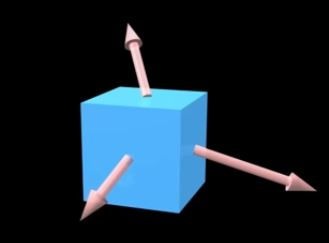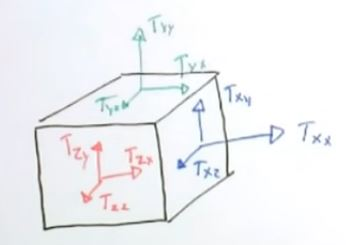There is one force for each orientation of the cube (x,y and z). This unit cube is often used to represent the local stress force at some specific point of a larger material: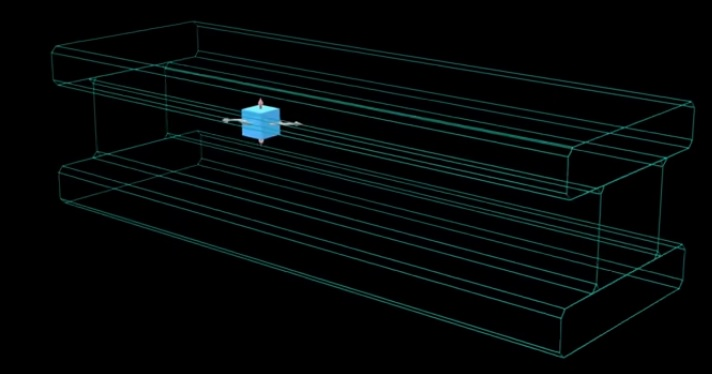Now what's particularly convenient with the stress Tensor T_{ij}, is that not only each row provides the stress force along x, y and z directions but we can infer the stress along any arbitrary direction $$\vec v$$. To do so we simply multiply the stress tensor by the desired direction:

$\vec v . T_{ij}$

In the case of our steel beam earlier we can slice it in a particular direction and infer the stress applied in the direction of that slice: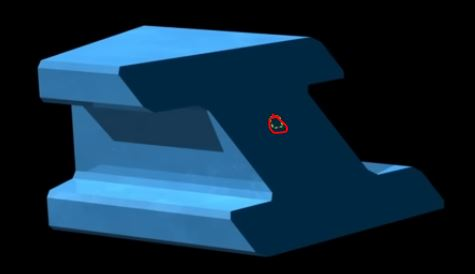Interestingly you can visualize the resulting stress forces by multiplying by every possible orientation, this give you a stress field: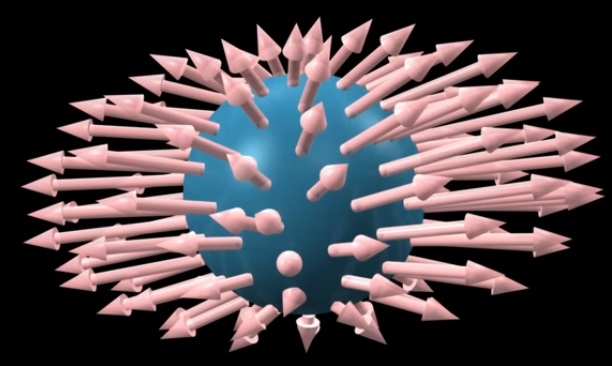Original animated explanations: Stress tensor visualization video

note: a property of the stress force applied to a material in state of static equilibrium (not moving or deforming), is that the unit cube should stay at equilibrium as well. This means the cube should not move rotate or otherwise deform if we consider all forces applied to it, they should all compensate each other (as Newton's 3rd law dictates). It results from that fact that the matrix representing a stress tensor should always be symmetrical.

# II - Invariant under coordinate change

The full definition of a tensor is that it has to be invariant under any change of coordinates. This rule means we should always be able to transform a tensor T to some other coordinate system so that the transformation T' still represents the same tensor. For instance the velocity vector should still point towards the same object whether we express its coordinates from some some place or another. Likewise we expect a stress tensor to deform an object the same way no matter the coordinate system we use to express it.
Illustration:Regardless of the coordinate system the pencil always points to the door with the same length. We can transform components with forward or backward transformations. (Note: if the vector/tensor becomes null under specific coordinate systems then we call it a pseudo-vector/tensor)

## III - Descriptive definition:

A rank-n tensor in m-dimensions is a mathematical object that has n indices and m^n components and obeys certain transformation rules.
or:
a tensor is a geometric object (vector, 3D reference frame) which is expressed and transformed according to a coordinate system defined by the user.

# IV- Alternate definitions

Tensor = a collection of vectors and covectors combined together using the tensor product. (kind of abstract/ recursive definition since you need to know about covectors and tensor product)

Alternate interpretation: Tensors as partial derivatives and gradients that transform with the Jacobian Matrix

# References

Good starter explanation

Others:

https://open.oregonstate.education/intermediate-fluid-mechanics/chapter/chapter-1-mathematical-tools/

ebook

https://gumroad.com/l/ubSc (it has a chapter on tensors)

What a tensor really is (French), must see